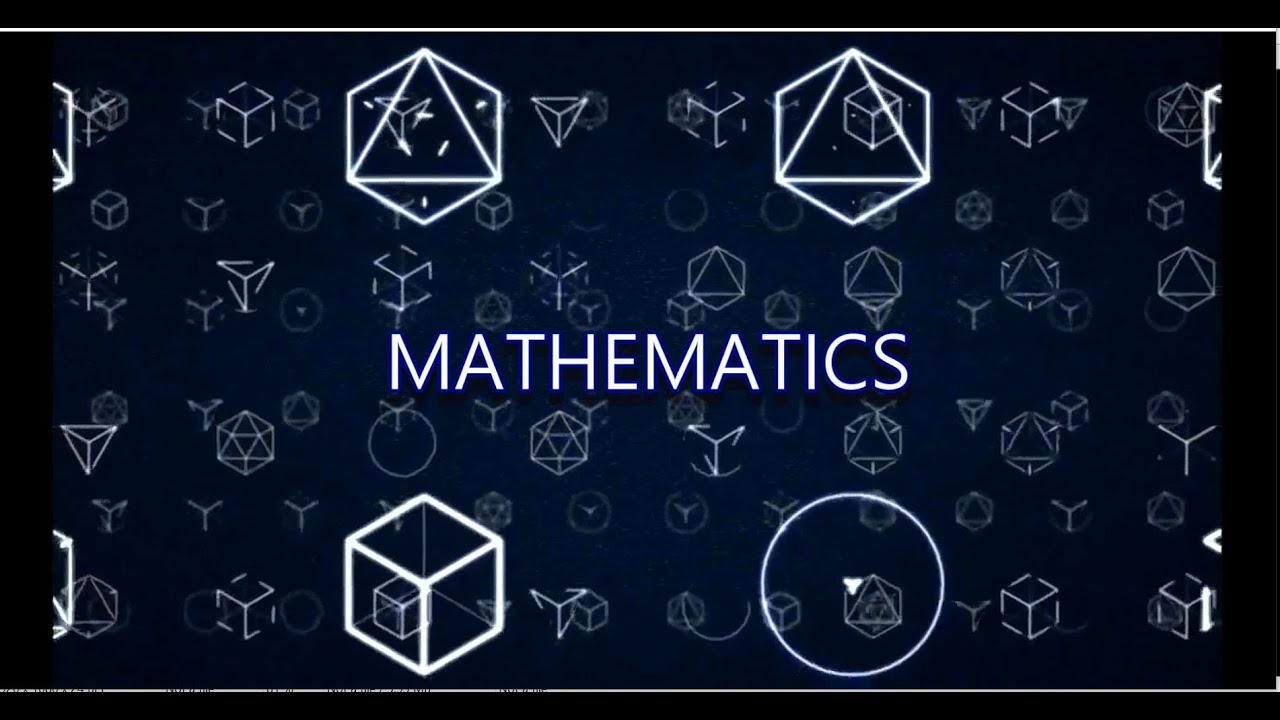# Mathematics

Mrs R Monk - Head of Department

Miss L Donegan - Assistant Head of Department

Mrs S Barlow

Mr N Gorman

Mr J Holliday

Miss P Lorains

Mr M Singh

Mr C Vaughton

Mathematics, rightly viewed, possesses not only truth, but beauty. Bertrand Russell

Mathematics is central to our understanding of the world in which we live; it underpins science, technology, medicine, economics and government.

OverviewThe mathematics classroom will provide students with the opportunity to see beyond the horizons set by the problems at hand. Our aim is for all students to enjoy mathematics and to achieve their potential. We use a variety of teaching styles to cater for their learning needs and are always available to support all students both in and out of the classroom. Lower School In the lower school students study the building blocks required for mathematics in the key areas of number, algebra, geometry and statistics.

An emphasis is also placed on problem solving and functional mathematics, to help students develop important skills that will be needed at GCSE and A Level. GCSE At GCSE, students study the AQA Linear specification, taking their GCSE examinations at the end of the course. The vast majority of our students take the higher examination, targeting grades 7, 8 and 9 (equivalent to the old A and A* grades).

In addition, our more able students also sit the AQA Level 2 Certificate in Further Mathematics. Advanced Level Mathematics A Level is a very popular subject in the Sixth Form and compliments numerous other subjects. Mathematics is demanding but also very enjoyable and rewarding. Our students will take the Edexcel A Level.

A number of students who are considering pursuing a Mathematics, Engineering, Computing or Economics degree also study the Edexcel Further Mathematics A Level. Extra-Curricular Activities Mathematics Clinic takes place once a week to support students from any year group with their mathematics.

In addition, a mentoring scheme has been introduced where Sixth Form mathematicians provide help to Key Stage 3 and 4 students who we feel would benefit from extra one-to-one support. Our strongest students take part each year in the National Maths Challenge which is run by the United Kingdom Mathematics Trust.

### KEY STAGE 5

Why A Level Mathematics?

Mathematics A Level is a demanding but rewarding course that is an ideal preparation for university study in a range of subjects including Medicine, Science, Engineering and of course, Mathematics itself. Studying Mathematics also complements other A Level courses such as Physics, Economics, Computing, Chemistry, Geography and Biology. Students who are good at Mathematics and enjoy the subject, consistently achieve very good grades at A Level Mathematics.

Even if your chosen course does not require Mathematics it is a very well respected A Level, demonstrating strong academic rigour, problem-solving, logical thinking and statistical analysis; all qualities highly valued by universities and employers for any career choice. Students will follow the Edexcel Mathematics specification.

Students will study the A Level course over two years, being assessed at the end of the course on both years’ course content. In the Lower Sixth, students will study a range of topics from the three mathematical areas of Pure Mathematics (including functions, graphs, equations, exponentials, logarithms, geometry, vectors, trigonometry and calculus), Statistics (including statistical sampling, measures of average and spread, the Binomial distribution, hypothesis testing and probability) and Mechanics (including kinematics, Newton’s Laws and forces). In Upper Sixth, students will study additional topics in Pure Mathematics (including sequences, parametric equations, numerical methods, further trigonometry, further calculus and differential equations), Statistics (including the Normal distribution, further hypothesis testing and further probability) and Mechanics (including vectors and further forces).

Further Mathematics

Further Mathematics has increased significantly in popularity in recent years. It tends to be a preferred A Level amongst the top Russell Group Universities for students considering a degree with a high mathematical content, such as, Mathematics, Engineering, Physics, Economics and Computing. Further Mathematics A Level can only be studied by students who are also studying Mathematics A Level. Students who are very good at Mathematics and work hard across the two years consistently achieve very good grades in both Mathematics A Level and Further Mathematics A Level because the two subjects complement each other.

Students will follow the Edexcel Further Mathematics specification. Students will study the A Level course over two years, being assessed at the end of the course on both years’ course content. In the Lower Sixth, students will study the mandatory Pure Mathematics module (which includes complex numbers, matrices, proof by induction and roots of equations) and two of four optional modules. Our students will study the optional Statistics module (which includes permutations, combinations, the Geometric distribution, the Poisson distribution, contingency tables, correlation and regression) and the optional Mechanics module (which includes dimensional analysis, work, energy, power, momentum, centre of mass and motion in a circle).

In Upper Sixth, students will study an additional mandatory module in Pure Mathematics (which includes summation of series, Hyperbolic functions, Maclaurin series, Polar co-ordinates and second order differential equations), and another two of four optional modules. Again, our students will study the optional Statistics module (which includes the Discrete Uniform distribution, continuous random variables, confidence tests and Normal approximations) and the optional Mechanics module (which includes rigid bodies, moments and linear motion under a variable force).